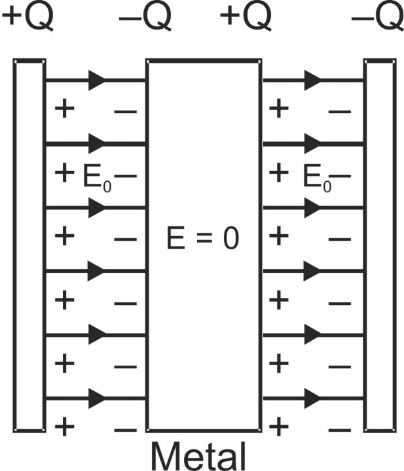# Electric lines of Force - Important points | Solved Examples

If we bring an electric charge into an electric field, how many places can we keep it? Infinitely many points, Right. As we know, it will experience a force which is different at different points. The problem is how can we represent the strength of the force at any point without calculating it mathematically, Michael Faraday came up with concept of imaginary lines, which are called as Electric lines of Force. They are also known as Electric Field Lines. Here you will get to know in detail about:

## Introduction

The idea of lines of force was introduced by Michael Faraday. A line of force is an imaginary curve the tangent to which at a point gives the direction of intensity at that point and the number of lines of force per unit area normal to the surface surrounding that point gives the magnitude of intensity at that point. The electric lines of force that represent the field of a positive electric charge in space consist of a family of straight lines radiating uniformly in all directions from the charge where they originate. A second positive charge placed in the field would travel radially away from the first charge. [source]

## Important Points on Electric lines of Force

1. Electric lines of force usually start or diverge out from positive charge and end or converge on negative charge. [caption id="attachment_4345" align="aligncenter" width="272"]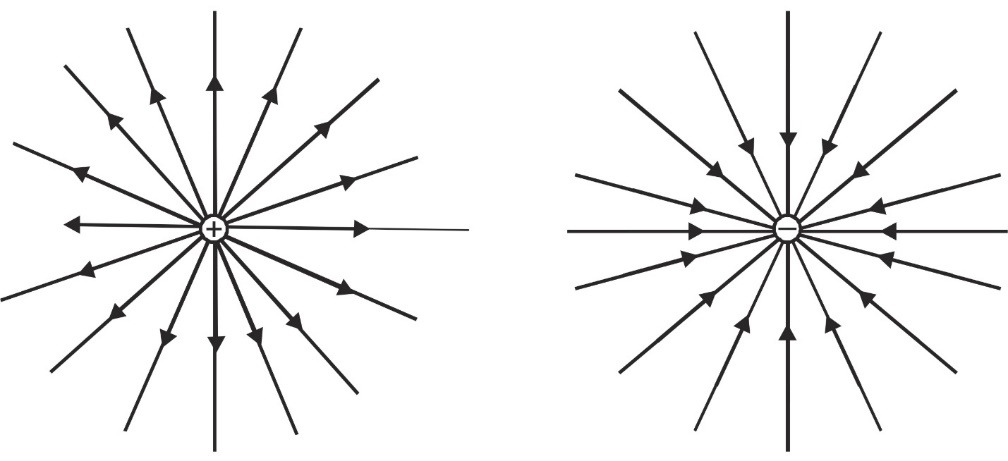Electric lines of force diverge from positive charge and converge in negative charge[/caption]
2. The number of lines originating or terminating on a charge is proportional to the magnitude of charge. In SI units 1/ε0 shows electric lines associated with unit (i.e., 1 coulomb) charge. So, if a body encloses a charge q, total lines of force or flux associated with it is q/ε0. If the body is cubical and charge is situated at its center the lines of force through each face will be q/6ε0. [caption id="attachment_4346" align="aligncenter" width="426"]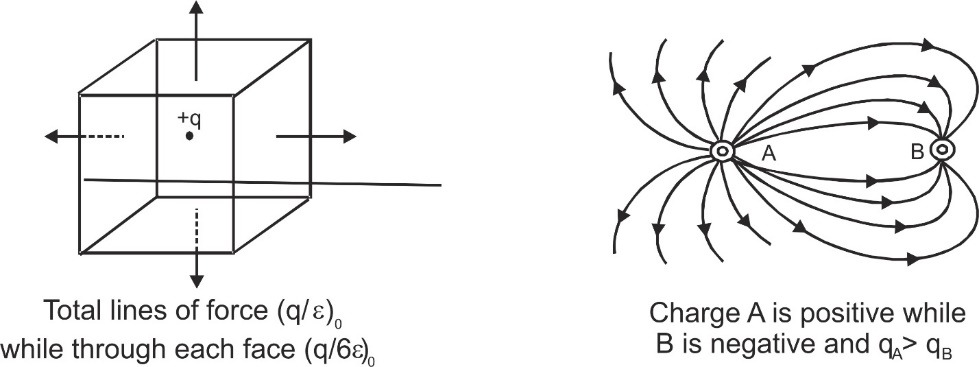Number of lines originating or terminating on a charge is proportional to the magnitude of charge[/caption]
3. Lines of force never cross each other because if they cross then intensity at that point will have two directions which is not possible.
4. In electrostatics, the electric lines of force can never be closed loops, as a line can never start and end on the same charge. If a line of force is a closed curve, work done round a closed path will not be zero and electric field will not remain conservative.
5. Lines of force have tendency to contract longitudinally like a stretched elastic string producing attraction between opposite charges and repel each other laterally resulting in, repulsion between similar charges and 'edge-effect' (curving of lines of force near the edges of a charged conductor). [caption id="attachment_4347" align="aligncenter" width="436"]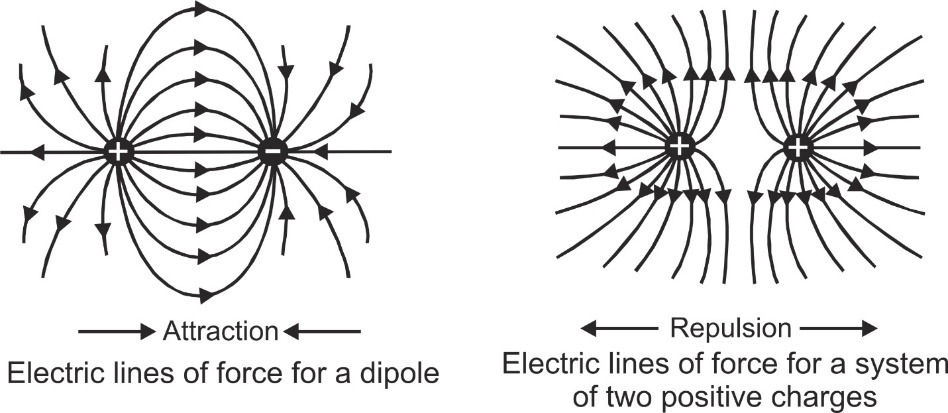Electric lines of Force for a Dipole & system of 2 Positive Charges[/caption]
6. If the lines of force are equidistant straight lines the field is uniform and if lines of force are not equidistant or straight lines or both, the field will be non-uniform. The first three represent non-uniform field while last shows uniform field. [caption id="attachment_4348" align="aligncenter" width="548"]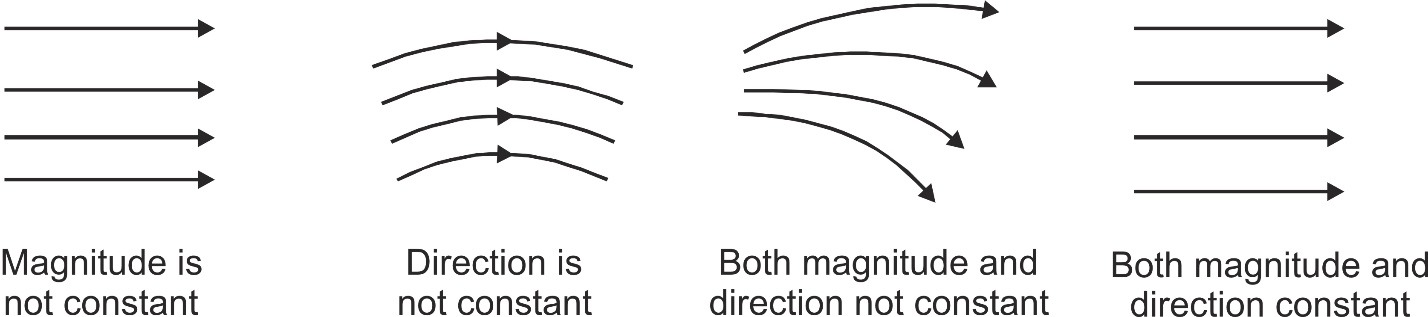Electric lines of force depending on Magnitude and Direction[/caption]
7. Electric lines of force end or start normally on the surface of a conductor. If a line of force is not normal to the surface of a conductor, electric intensity will have a component along the surface of the conductor and hence conductor will not remain equipotential which is not possible as in electrostatics conductor is an equipotential surface. [caption id="attachment_4349" align="aligncenter" width="536"]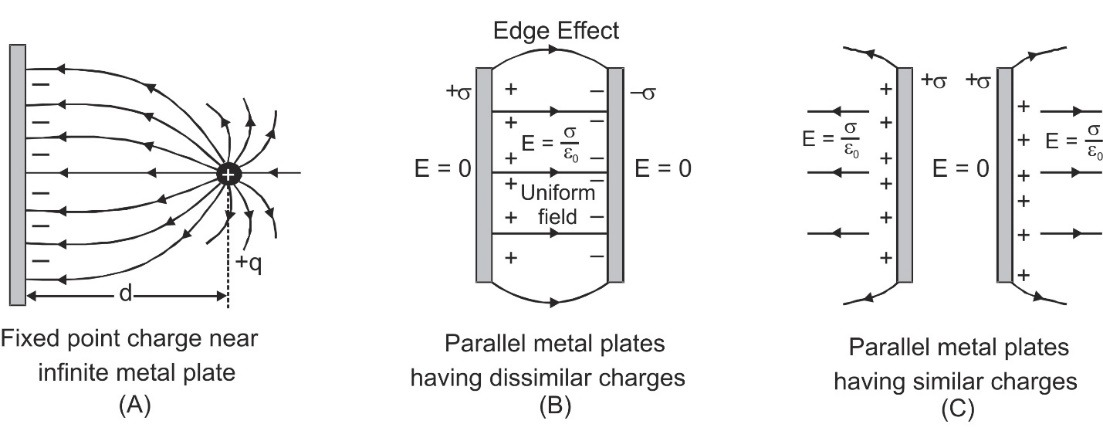Edge Effect[/caption]
8. If in a region of space, there is no electric field there will be no lines of force. This is why inside a conductor or at a neutral point where resultant intensity is zero there is no line of force.
9. The number of lines of force per unit normal area at a point represents magnitude of electric field intensity. The crowded lines represent strong field while distant lines show a weak field.
10. The tangent to the line of force at a point in an electric field gives the direction of intensity. It gives direction of force and hence acceleration which a positive charge will experience there (and not the direction of motion). A positive point charge free to move may or may not follow the line of force. It will follow the line of force if it is a straight line (as direction of velocity and acceleration will be same) and will not follow the line if it is curved as the direction of motion will be different from that of acceleration. The particle will not move in the direction of motion or acceleration (line of force) but other than these which will vary with time as $\mathop v\limits^ \to = \mathop u\limits^ \to + \mathop {at}\limits^ \to$

## Comparison of Electric and Magnetic lines of Force

1. Electric lines of force never form closed loops while magnetic lines of force are always closed loops.
2. They always emerge or terminate normally on the surface of a charged conductor, while magnetic lines of force start or terminate on the surface of a magnetic material at any angle.
3. Electric lines of force do not exist inside a conductor but magnetic lines of force may exist inside magnetic material.
4. Total electric lines of force linked with a closed surface may or may not be zero but total magnetic lines of force linked with a closed surface is always zero (as mono-poles do not exist).

## Solved Examples

Q. A solid metallic sphere is placed in a uniform electric field. Which of the lines A, B, C and D shows the correct path and why?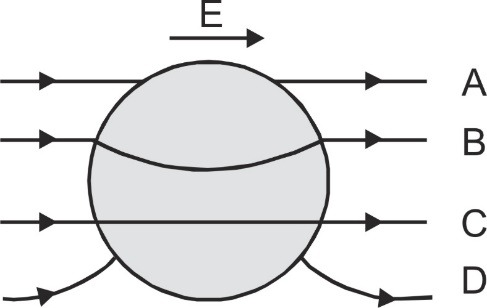Ans. Path (A) is wrong as lines of force do not start or end normally on the surface of a conductor. Path (B) and (C) are wrong as lines of force should not exist inside a conductor. Also, lines of force are not normal to the surface of conductor. Path (D) represents the correct situation as here line of force does not exist inside the conductor and starts and ends normally on its surface.
Q. A metallic slab is introduced between the two plates of a charged parallel plate capacitor. Sketch the electric lines of force between the plates.
Ans. Keeping in mind the following properties:
1. Lines of force start from positive charge and end on negative charge.
2. Lines of force start and end normally on the surface of a conductor.
3. Lines of force do not exist inside a conductor (as field inside a conductor is zero) The field between the plates is shown as